# Basic Geometry : How to find if right triangles are similar

## Example Questions

2 Next →

### Example Question #11 : How To Find If Right Triangles Are Similar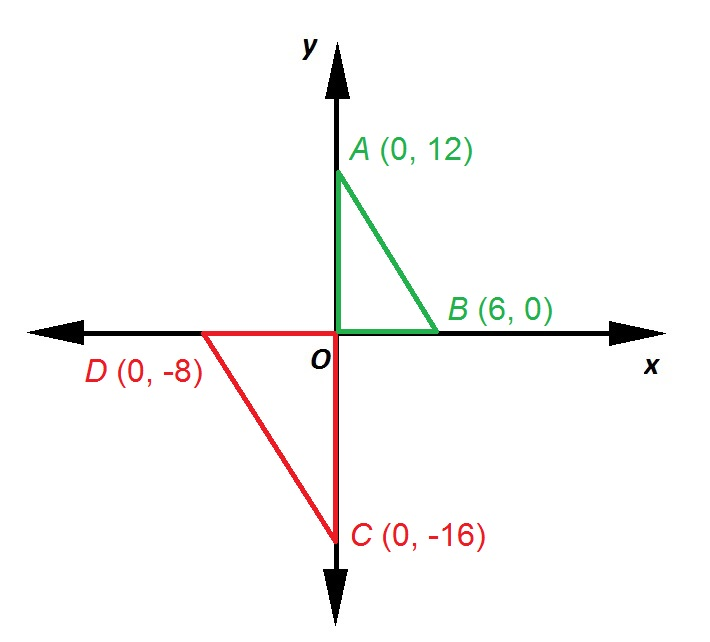Refer to the above diagram.

True or false: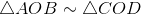False

True

True

Explanation:

The distance from the origin to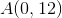is the absolute value of the-coordinate of, which is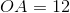. Similarly,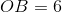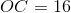, and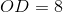. Also, since the axes intersect at right angles,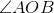and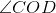are both right, and, consequently, congruent.

According to the Side-Angle-Side Similarity Theorem (SASS), if two sides of a triangle are in proportion to the corresponding sides of a second triangle, and their included angles are congruent, the triangles are similar.

We can test the proportion statement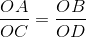by substituting: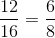Test the truth of this statement by comparing their cross products: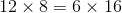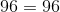The cross-products are equal, making the proportion statement true, so two pairs of sides are in proportion. Also, their included anglesandare congruent. This sets up the conditions of SASS, so.

### Example Question #12 : How To Find If Right Triangles Are Similar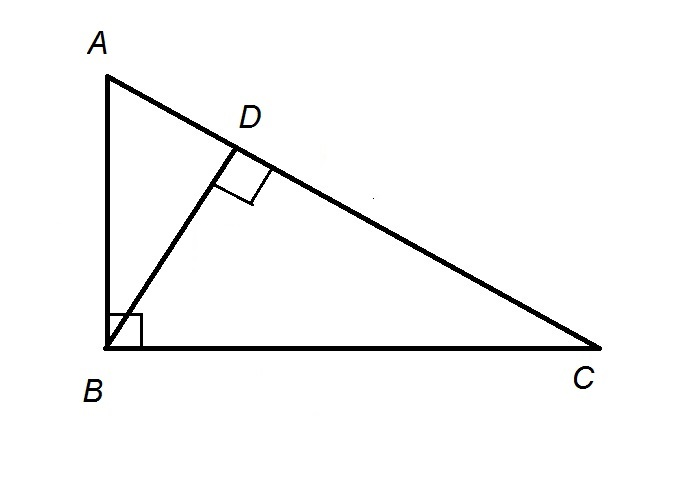Refer to the above figure.

True, false, or inconclusive: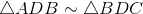.

True

Inconclusive

False

True

Explanation: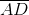is an altitude of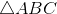, so it divides the triangle into two smaller triangles similar to each other - that is, if we match the shorter legs, the longer legs, and the hypotenuses, the similarity statement is.

2 Next →

### All Basic Geometry Resources